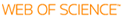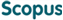### BROWSE

Deep Neural Networks to Learn Basis Functions with a Temporal Covariance Loss

Cited 0 times inCited 0 times inTitle
Deep Neural Networks to Learn Basis Functions with a Temporal Covariance Loss
Author
Issue Date
2019-08
Publisher
Abstract
Deep Neural Networks (DNNs) and Gaussian Processes (GPs) are commonly used prediction models to solve regression problems on time series data. A GP can approximate a smooth function arbitrarily well. When the function satisfies some conditions. We adopt the principles of GP learning to DNN learning on time series data. While previous approaches need to change the architecture of DNNs or be explicitly derived from the GPs algorithm, we concentrate on the learning scheme of DNNs to leverage the important principles of GPs by proposing the Temporal Covariance loss function. Whereas the conventional loss function of DNNs only captures the mean of the target values, Temporal Covariance loss function further captures the covariance of the target values where covariance function is the other factor to define GPs along with the mean function. We show that learning DNNs and Convolutional Neural Networks (CNNs) with the Temporal Covariance loss function can obtain more accurate models for sets of regression problems with US groundwater data and NASDAQ 100 stock data.
Description
Department of Computer Science and Engineering
URI